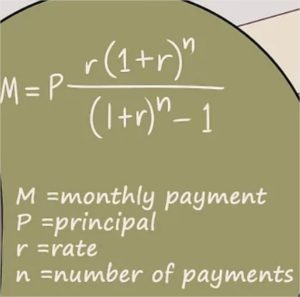# Java Mortgage Payment Calculator in 3 Ways | Java Programs

Java Program To Calculate Mortgage Payment – The following java code has been written in 3 simple ways for mortgage payment calculator. If you have any queries leave a comment here.

• Using Standard Method
• Using Static Method
• Using Separate ClassMortgage Payment, as we all know, is the amount of money that you need to return to the person or entity where you have your property mortgaged with.

The frequency of the transaction is monthly and can be calculated with the help of a simple formula.

The formula for Mortgage Payment is as follows:

M = P [{r(1+r)^n}/{(1+r)^n – 1}]

where

M = Monthly payment

P = principal

r = rate

n = number of payments

With the help of this formula, you can easily calculate the amount of money that you need to give back.

Thus, the various methods to calculate mortgage payment in Java Programming are as follows:

## Java Mortgage Payment

One of the basic way every beginner codes is that, they write the entire program in the main method of the program. This is the standard method that people generally follow.

So, initially our inputs are read at run time using the scanner class.

Our problem requires principal amount (principal), rate of interest (rate), time period (time).  The input rate that we have taken is in the form of percentage rate per the entire year, but we require this on a monthly basis. So, we make use of the below formula.

Apart from rate, even the time period is taken in years, so we convert years into months using,

After getting all the desired quantities for performing the calculation for the monthly payment, we make use of the standard formula used i.e.,

double p= (principal * rate) / (1 – Math.pow(1 + rate, -time));

To perform power function in Java, we make use of the Math package which consists of the pow method in it.  The math pow function returns a double as is syntactically represented as Math.pow(base,exponent).

The end result stored in p is the payment that is required to be made which can be printed in the console screen.

Output:

### Mortgage Java – Static Method

Static method is a separate method apart from main which doesn’t need an object to be created to reference it and usually makes use of static data variables.

In the standard method we have observed that, the entire code being written in the same main method.

This is not very efficient when it comes to readability of the code. So, to make it more convenient, we make use of another separate static method.

Here, the main method only comprises of the required inputs taken using the scanner class along with the basic calculations like:

Apart from this, the essential algebraic equation used for getting the mortgage payment is written in the static method (payment) which returns a value of type double which gives the payment to be made.

Output:

Using Separate Class

In the above scenario of static method we have seen that using another static method we increase the readability of the code. There is another way to enhance it further and that is by making use of a separate class itself for logic.

When the logic is written in a completely different class not just does it increase the readability and scalability but also, each class would not be aware of the contents in another class so it enhances the security of data as well.

So a separate class (Payment) can be created consisting of method which has the logic behind getting the payment i.e.,

while, the main method takes in input with help of the scanner class at run time and instantiates an object referring to this separate class (Payment) with which the method consisting of the logic (payment) can be invoked.

Output1:

x

## Java Program to Add Two Matrices – 4 Ways | Programs

Java program to add two matrices – The following Java Code will let you know ...